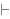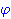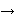Intuitionistic Logic Explorer < Previous   Next > Nearby theorems Mirrors  >  Home  >  ILE Home  >  Th. List  >  loowoz Unicode version

Theorem loowoz 100
 Description: An alternate for the Linearity Axiom of the infinite-valued sentential logic (L-infinity) of Lukasiewicz, due to Barbara Wozniakowska, Reports on Mathematical Logic 10, 129-137 (1978). (Contributed by O'Cat, 8-Aug-2004.)
Assertion
Ref Expression
loowozProof of Theorem loowoz
StepHypRef Expression
1 jarr 95 . 221a2d 26 1Colors of variables: wff set class Syntax hints:wi 4 This theorem was proved from axioms:  ax-1 5  ax-2 6  ax-mp 7 This theorem is referenced by: (None)
 Copyright terms: Public domain W3C validator